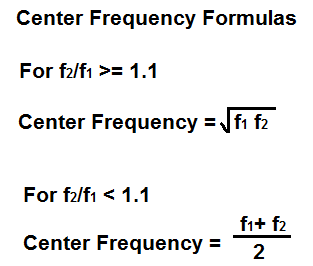﻿ Center Frequency Calculator ﻿# Center Frequency CalculatorEnter the Lower Cutoff Frequency Hz (hertz) KHz (kilohertz) MHz (megahertz) Enter the Upper Cutoff Frequency Hz (hertz) KHz (kilohertz) MHz (megahertz)

Center Frequency:

﻿

The center frequency calculator calculates the center frequency of either a bandpass filter or the center frequency of notch filter, which is also referred to as the notch frequency or the null frequency.

The center frequency represents the midpoint frequency in between the -3dB cutoff frequencies of a bandpass or notch filter. The -3dB cutoff points are also referred to as the lower cutoff frequency and upper cutoff frequency of a filter circuit.

For a bandpass filter, the center frequency and frequencies in close proximity to it are where there will be maximum gain in the passband. It is part of the passband that has maximum gain. On the other hand, the -3dB cutoff points do not have maximum gain. They have a -3dB drop in gain, which represents 0.707V of the maximum voltage of the filter circuit.

Generally, the center frequency is calculated by the square root of the product of the 2 cutoff frequencies. The formula is shown by, fcenter= √  f1 f2

However, for narrowband bandpass filters where the ratio of f2/f1 is less than 1.1, the center frequency can be approximated by the addition of the 2 cutoff frequencies divided by 2. This is shown in the formula, fcenter= (f1 + f2)/2.

For a notch, or bandstop filter, the center frequency is also referred to as the null frequency or the notch frequency. The null frequency represents the midpoint in the stopband in which there is maximum attenuation of the signal. This point exists in between the -3dB cutoff frequencies, just like with a bandpass filter. To see a visual of the center frequency of a notch filter against a bandpass filter, see Center Frequency of Bandpass and Notch Filters.

So this calculator is perfectly applicable to either bandpass filters or notch filters.

To use this calculator, simply enter the lower cutoff frequency and the upper cutoff frequency. The center frequency will be then be calculated and displayed.

The center frequency is very useful not only because it shows the midpoint of the cutoff frequencies, thus showing the ideal middle spot of maximum gain, but it's also useful because it is used to calculate other useful metrics for either bandpass or notch filter circuits. For example, the center frequency is used to calculate the quality factor of a bandpass or notch filter circuit. For a bandpass filter, the quality factor is the ratio of the center frequency of the bandpass over the entire bandpass region from the lower to upper cutoff frequencies. Therefore, for a bandpass filter, the quality factor, Q= fcenter/ (f2 - f1). The quality factor shows how narrow or wide the passband is for a bandpass filter. For a notch filter circuit, the quality factor is defined as the ratio of the entire stopband from f2 to f1 over the center frequency of the stopband, which is referred to as the null frequency or notch frequency. So, for a notch filter, the quality factor is the entire stopband from the -3dB cutoff points over the null frequency, where the attenuation is the greatest. The quality factor of a notch filter is, Q= (f2 - f1)/fcenter. The quality factor shows how narrow or wide the stopband is for a notch filter. So this is an important metric for dealing with certain type of filters that requires the calculation of a center frequency. A high quality factor indicates the passband or stopband is narrow, while a low quality factor indicates the passband or stopband is wide.

Related Resources

﻿